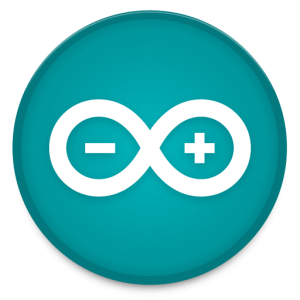# Arduino Beginner’s Course Lesson 2– Introduction to Arduino part 2

In this lesson we will go through the Arduino language. We’ll cover programming basics and introduce some of the libraries provided by Arduino. The Arduino Language is very similar to C. It’s almost the same language but Arduino provides us with several libraries to make things a bit easier. If you have absolutely no knowledge of any programming language I suggest you learn basic C programming. It will become very useful in the future and we cannot cover C programming here! 🙂

Basic Stuff
In the first lesson Introduction to Arduino we have introduced a very simple sketch that blinks an LED on and off for a period of 1 second. I hope you had some time to change the values to see what happens 🙂

Now it’s time to explain the code and introduce some interaction with our little LED.
``` int ledPin = 13; ```

This line initializes an int variable named ledPin with value 13. This will be used to specify the digital pin on the Arduino board. Normally pin 13 has an LED attached to it, so we should see something somewhere blink 🙂

``` void setup() { pinMode(ledPin,OUTPUT); } ```

We already mentioned a little about the setup() function, it is required by the Arduino platform. Inside this function we put the code that needs to run first and only once, after the Arduino is restarted.

pinMode is the function to set a pin into OUTPUT or INPUT mode (Note: keywords are all caps). To write to a pin we use the OUTPUT, to read a pin’s value we use INPUT. In this case we need to switch an LED on/off so we will be writing to the pin hence OUTPUT is used.

``` void loop(){ digitalWrite(ledPin,HIGH); delay(1000); digitalWrite(ledPin,LOW); delay(1000); } ```

The loop() function is the main function of the sketch. It basically runs all the time in a continuous manner. When it reaches the bottom, it starts over again. The digitalWrite function takes 2 arguments the first is the pin number, in our case it’s 13, the second argument is the value to be assigned, HIGH or LOW. When setting the pin to HIGH, 5v pass through the pin, when set to low the pin is grounded meaning it will give us a reading of 0v hence when putting the pin to LOW, the LED turns off.
The delay function pauses the execution of the sketch for a number of milliseconds, 1 second = 1000 milliseconds.
That settled, we can dive a little bit deeper into Arduino. We will introduce an internal library  which is used to communicate directly to the PC via the USB cable (finally something interesting 🙂 ). The library is called Serial, which basically implements a lot of complex algorithms to communicate over the USB port in a very simple way. In the setup function we need to add``` ```

``` Serial.begin(9600); ```

This function will initialize the serial communication with a speed of 9600 bits per second, the normal speed used to share text data over serial. In the loop function we need to write something to the PC.

``` Serial.println("LED is ON"); Serial.println("LED is OFF"); ```

These two lines will send the text “LED is ON” and “LED is OFF” respectively, to the PC. We’ll incorporate this into our original code and it should look something like this

``` int ledPin = 13; ```

```void setup() { Serial.begin(9600); pinMode(ledPin,OUTPUT); } void loop(){ digitalWrite(ledPin,HIGH); Serial.println("LED is ON"); delay(1000); digitalWrite(ledPin,LOW); Serial.println("LED is OFF"); delay(1000); } ```

Upload the sketch using the upload button (the arrow button). Now we need a Serial client to read the text being sent by the Arduino. The IDE already provides that. On the top right corner there is an icon which resembles a lens. If you click on it, the serial monitor will pop up. As soon as you do, text will start lining up in this manner

`LED is ON`

`LED is OFF`

`LED is ON`

We can send everything via serial. For example we can send integers, bytes, or a byte array. We will go through these in the lessons to come.

From now on you should be able to understand a little Arduino programming. Feel free to explore somethings on your own. Try not to burn down your Arduino though.

Go to Lesson 3 here